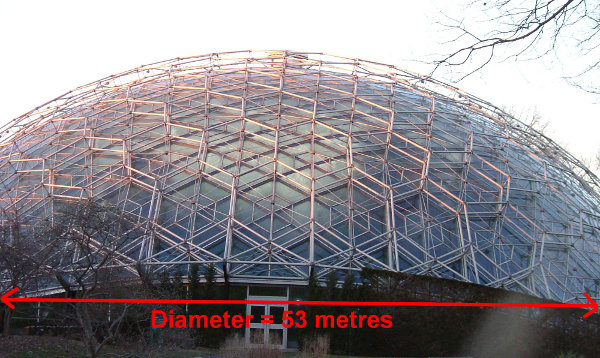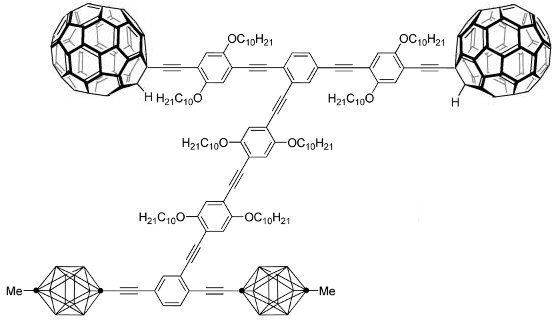# Surface Area Of Sphere

Surface Area of Sphere = 4 π r2

## Maths Fun - Surface Area of an Orange

You will need an orange, a knife, sticky tape and a ruler. Cut the orange in half and lay it with the flat side down. Tape the sticky tape from the top of the orange and go around and around the orange until it is covered with one layer of tape. Don't overlap. Now undo the tape and measure its length and its width. Calculate the area of the tape you used.

Now measure the diameter of the orange. Use the rule for SA of Sphere to work out the surface area of the orange. Compare your two calculations.

## Example One - Surface Area of a SphereWhat is the area of plastic of a basketball with a diameter of 24 cm?
(Use π = 3.14. Take care to use the radius.)

Answer:
SA of sphere
= 4 π r2
= 4 × 3.14 × 12 × 12
= 1808.64 cm2

## Example Two - Surface Area of a HemisphereIn the Missouri Botanic Gardens in USA is a geodesic dome (almost a hemisphere). Its diameter is 53 metres. What is the approximate area of perspex (acrylic)? (Use π = 3.14.)

Answer:
SA of hemisphere
= (4 π r2) / 2
= (4 × 3.14 × 26.5 × 26.5) / 2
= 4410.13 m2

## Maths Fun - Super Soap Bubbles RecipeMix 5% detergent, nearly 50% glycerine (from the pharmacy) and nearly 50% water. Dip a wire loop in the mixture and blow!

## Challenge Question - Surface Area to Volume Ratio

There are many objects containing gas and liquid that have an efficient spherical shape. Consider the shapes of body cells and bubbles.

Design and carry out an activity that shows that:

• Circles are the most efficient shapes to have the greatest area for the same perimeter.
• Spheres are the most efficient shape to have the largest volume for the same surface area. (Volume of Sphere = 43 π r3)

## Did You Know That...?This is a Nano-dragster using Bucky Balls for wheels. Nanotechnology has many functions from nano[ro]bots which can clear blocked blood vessels and delivering medication to targeted body cells, to super-conductors.